• python机器学习——正则化
2020-12-05 14:15:52

我们在训练的时候经常会遇到这两种情况：

1、模型在训练集上误差很大。

2、模型在训练集上误差很小，表现不错，但是在测试集上的误差很大

我们先来分析一下这两个问题：

对于第一个问题，明显就是没有训练好，也就是模型没有很好拟合数据的能力，并没有学会如何拟合，可能是因为在训练时我们选择了较少的特征，或者是我们选择的模型太简单了，不能稍微复杂的拟合数据，我们可以通过尝试选取更多的特征、增加一些多项式特征或者直接选用非线性的较复杂的模型来训练。

对于第二个问题，可以说是第一个问题的另外一个极端，就是模型对训练集拟合的太好了，以至于把训练集数据中的那些无关紧要的特征或者噪音也学习到了，导致的结果就是当我们使用测试集来评估模型的泛化能力时，模型表现的很差。打个不恰当比方就是你平时把作业都背下来了，但是其实你并没有学会如何正确解题，所以遇到考试就考的很差。解决方法就是增加训练集的数据量或者减少特征数量来尝试解决。

第一个问题我们叫做欠拟合(underfitting)，第二个问题我们叫做过拟合(overfitting)这两个问题还存在一种解决方法，就是我接下来要说的正则化。

我们之前说模型学习的过程也就是调整权重参数的过程，通过训练集中的数据来将模型的权重参数调整到一个使得损失函数最小的值。

对于一个分布较复杂的样本，如果训练得到的权重参数维度太少或者参数过小，也就是特征项很少，一些重要的特征没有起到作用，那么这条拟合曲线就会变得很简单，我们看上图的欠拟合图像，里面的拟合曲线是一条直线，这就是权重参数维度太少的结果。而如果权重参数维度过多或者参数过大，导致拟合曲线过于复杂，如上图的过拟合图像，拟合曲线可以完美的将两类不同的样本点区分开，但是我们也可以看出这条曲线很复杂，权重参数的项数一定很多。

现在进入正题，对于正则化，我们常见的形式是L2正则：

$\frac \lambda 2\lVert w \lVert^2 = \frac \lambda 2 \sum_{j=1}^m{w_j^2}$

这里的

$\lambda$

就是正则化系数。

我们将正则项直接添加到损失函数后即可使用，比如对于逻辑回归模型，带有L2正则项的损失函数为：

$J(w)=\sum_{i=1}^n\left[-y^{(i)}log(\phi(z^{(i)}))-(1-y^{(i)})log(1-\phi(z^{(i)}))\right] + \frac \lambda 2\lVert w \lVert^2$

我们通过控制正则化系数来控制权重参数的大小。一般正则化用于解决模型过拟合的问题，我们的训练目标是为了使损失函数最小，但是如果权重参数过大会导致过拟合，模型泛化能力下降，那么为了解决这个问题，将正则项加到损失函数后面，组成一个新的损失函数，为了最小化这个新的损失函数，我们在训练过程中不仅要使得误差小，还要保证正则项不能太大，于是如果我们选择一个较大的正则化系数，那么为了保证正则项不能太大，就会使得权重参数变小，这也就是我们的最终目的：在保证误差不大的情况下，使得权重参数也不能太大，缓解了过拟合问题。正则化系数越大，正则化越强，权重参数越小。

所以对于欠拟合的模型，我们也可以尝试减小正则化系数来增大权重参数，而对于过拟合模型，我们尝试增大正则化系数来减小权重参数。

更多相关内容
• 正则化的详细解释请参考： https://www.bilibili.com/video/BV1aE411L7sj?p=3 https://zhuanlan.zhihu.com/p/29957294 代码 from sklearn import preprocessing x = [[1, 2, 3], [50, 1, 2], [3, 0, -10]] x_...

正则化的详细解释请参考：

# 代码

from sklearn import preprocessing

x = [[1, 2, 3],
[50, 1, 2],
[3, 0, -10]]
x_normal = preprocessing.normalize(x)
print(x_normal)


结果是：

[[ 0.26726124  0.53452248  0.80178373]
[ 0.9990015   0.01998003  0.03996006]
[ 0.28734789  0.         -0.95782629]]


# 参考文档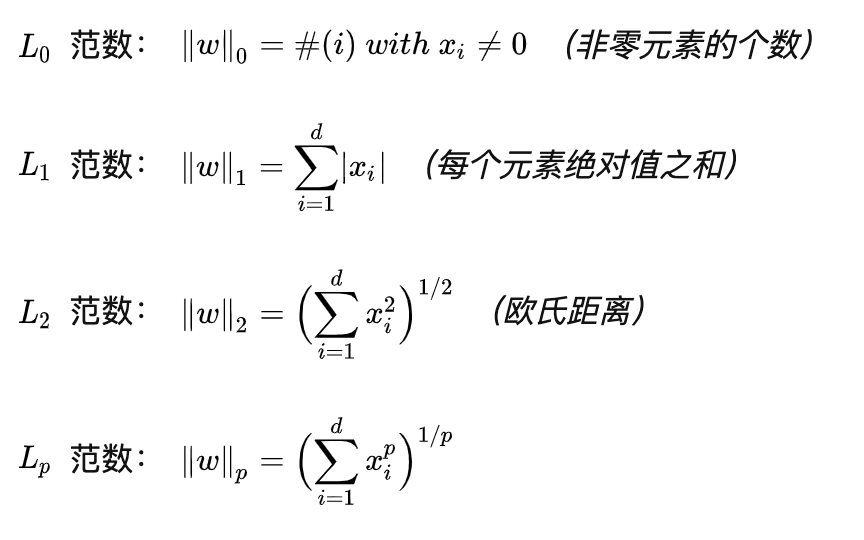展开全文• 于是便有了正则化方法的出现，通过收缩方法(正则化方法)进行回归。正则化方法主要包括岭回归与LASSO回归。/ 01 / 岭回归岭回归通过人为加入的惩罚项(约束项)，对回归系数进行估计，为有偏估计。有偏估计，允许估计有...接之前的线性回归文章，传送门如下。

上面这篇文章是利用方差膨胀因子，去诊断与减轻多重共线性对线性回归的影响。

需要人为介入(根据得到的方差膨胀值去判断)，耗费过多的时间。

于是便有了正则化方法的出现，通过收缩方法(正则化方法)进行回归。

正则化方法主要包括岭回归与LASSO回归。

/ 01 / 岭回归

岭回归通过人为加入的惩罚项(约束项)，对回归系数进行估计，为有偏估计。

有偏估计，允许估计有不大的偏度，以换取估计的误差显著减小，并在其残差平方和为最小的原则下估计回归系数。

通常岭回归方程中的R²会稍低于线性回归分析，但回归系数的显著性往往明显高于普通线性回归。

这里不对相应的理论知识进行细说，说实话小F也是晕乎乎...

所以选择先调包，看看效果是啥样的。

使用机器学习框架scikit-learn进行岭回归参数的选择(正则化系数)。

数据是书中的数据，已上传网盘，公众号回复「正则化」，即可获取。

scikit-learn当中的模型不会默认对数据标准化，必须手动执行。

标准化后的数据可以消除量纲，让每个变量的系数在一定意义下进行直接比较。

import numpy as np

import pandas as pd

import matplotlib.pyplot as plt

from sklearn.linear_model import Ridge

from sklearn.linear_model import RidgeCV

from sklearn.preprocessing import StandardScaler

# 消除pandas输出省略号情况及换行情况

pd.set_option('display.max_columns', 500)

pd.set_option('display.width', 1000)

# 读取数据,skipinitialspace:忽略分隔符后的空白

# 获取信用卡有支出的行数据

exp = df[df['avg_exp'].notnull()].copy().iloc[:, 2:].drop('age2', axis=1)

# 获取信用卡无支出的行数据,NaN

exp_new = df[df['avg_exp'].isnull()].copy().iloc[:, 2:].drop('age2', axis=1)

# 选择4个连续变量,分别是年龄 收入 当地小区价格 当地人均收入

continuous_xcols = ['Age', 'Income', 'dist_home_val', 'dist_avg_income']

# 标准化

scaler = StandardScaler()

# 解释变量,二维数组

X = scaler.fit_transform(exp[continuous_xcols])

# 被解释变量,一维数组

y = exp['avg_exp_ln']

# 生成正则化系数

alphas = np.logspace(-2, 3, 100, base=10)

# 使用不同的正则化系数对模型进行交叉验证

rcv = RidgeCV(alphas=alphas, store_cv_values=True)

# 使用数据集训练(fit)

rcv.fit(X, y)

# 输出最优参数,正则化系数及相应模型R²

print('The best alpha is {}'.format(rcv.alpha_))

print('The r-square is {}'.format(rcv.score(X, y)))

# 训练好后使用transform进行数据转换

X_new = scaler.transform(exp_new[continuous_xcols])

# 使用模型对数据做预测

print(np.exp(rcv.predict(X_new)[:5]))

输出结果如下。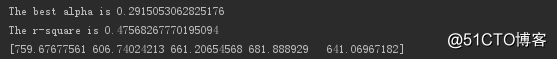最优正则化系数为0.29，模型R²为0.475。

并使用最优正则化系数下的岭回归模型预测数据。

对不同正则化系数下模型的均方误差进行可视化。

# 正则化系数搜索空间当中每轮交叉验证的结果,模型的均方误差

cv_values = rcv.cv_values_

n_fold, n_alphas = cv_values.shape

# 模型均方误差上下波动值

cv_mean = cv_values.mean(axis=0)

cv_std = cv_values.std(axis=0)

ub = cv_mean + cv_std / np.sqrt(n_fold)

lb = cv_mean - cv_std / np.sqrt(n_fold)

# 绘制折线图,x轴是指数型形式

plt.semilogx(alphas, cv_mean, label='mean_score')

# y1(lb)和y2(ub)之间进行填充

plt.fill_between(alphas, lb, ub, alpha=0.2)

plt.xlabel('$\\alpha$')

plt.ylabel('mean squared errors')

plt.legend(loc='best')

plt.show()

输出结果如下。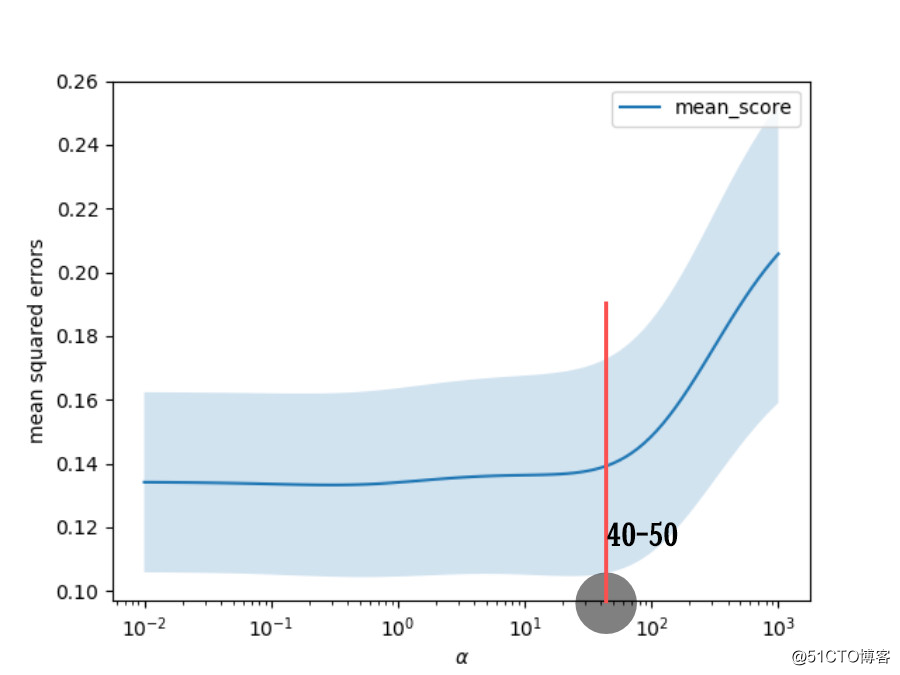发现正则化系数在40或50以下时，模型的均方误差相差不大。

当系数超过该阈值时，均方误差则快速上升。

所以正则化系数只要小于40或50，模型的拟合效果应该都不错。

正则化系数越小则模型拟合越好，但过拟合情况也越容易发生。

正则化系数越大，则越不容易过拟合，但模型的偏差越大。

RidgeCV通过交叉验证，可以快速返回“最优”的正则化系数。

当这只是基于数值计算的，可能最终结果并不符合业务逻辑。

比如本次模型的变量系数。

# 输出模型的变量系数

print(rcv.coef_)

# 输出结果

[ 0.03321449 -0.30956185  0.05551208  0.59067449]

发现收入的系数为负值，这肯定是不合理的。

下面通过岭迹图进行进一步分析。

岭迹图是在不同正则化系数下变量系数的轨迹。

ridge = Ridge()

coefs = []

# 不同正则化系数下的变量系数

for alpha in alphas:

ridge.set_params(alpha=alpha)

ridge.fit(X, y)

coefs.append(ridge.coef_)

# 绘制变量系数随正则化系数变化的轨迹

ax = plt.gca()

ax.plot(alphas, coefs)

ax.set_xscale('log')

plt.xlabel('alpha')

plt.ylabel('weights')

plt.title('Ridge coefficients as a function of the regularization')

plt.axis('tight')

plt.show()

输出结果。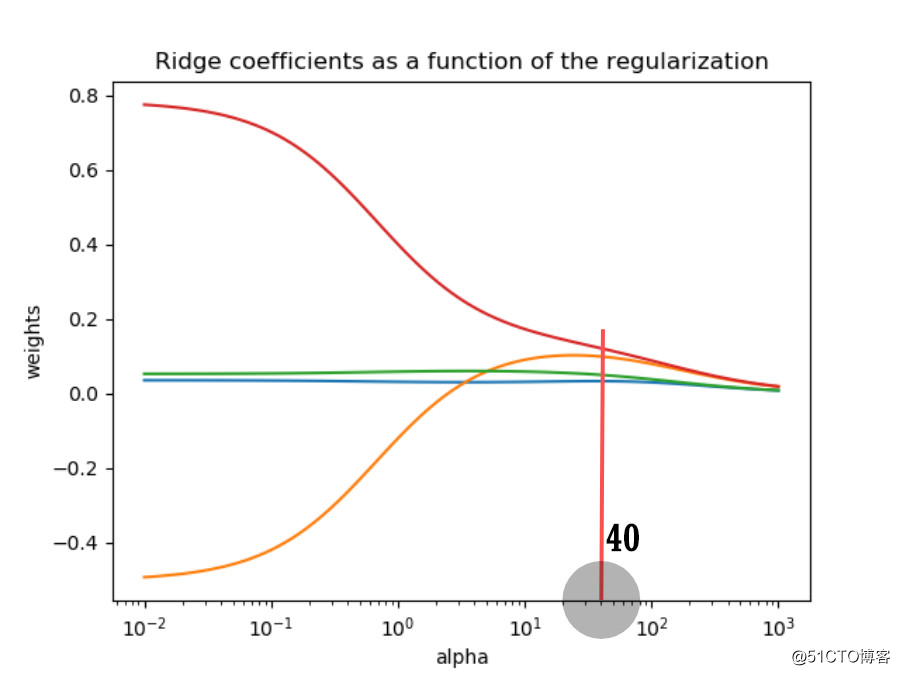①有两个变量的系数在不同的正则化系数下都很接近于0，那么可以选择删除。

②正则化系数越大，对变量系数的惩罚越大，所有变量的系数都趋近于0。

③有一个变量的系数变化非常大(有正有负)，说明该系数的方差大，存在共线性的情况。

综合模型均方误差和岭迹图的情况，选取正则化系数为40。

如果大于40，则模型均方误差增大，模型拟合效果变差。

如果小于40，则变量系数不稳定，共线性没有得到抑制。

那么就来看看，当正则化系数为40时，模型变量系数的情况。

ridge.set_params(alpha=40)

ridge.fit(X, y)

# 输出变量系数

print(ridge.coef_)

# 输出模型R²

print(ridge.score(X, y))

# 预测数据

print(np.exp(ridge.predict(X_new)[:5]))

# 输出结果

[0.03293109 0.09907747 0.04976305 0.12101456]

0.4255673043353688

[934.79025945 727.11042209 703.88143602 759.04342764 709.54172995]

发现变量系数都为正值，符合业务直觉。

收入和当地人均收入这两个变量可以保留，另外两个删除。

/ 02/ LASSO回归

LASSO回归，在令回归系数的绝对值之和小于一个常数的约束条件下，使残差平方和最小化。

从而能够产生某些严格等于0的回归系数，得到解释力较强的模型。

相比岭回归，LASSO回归还可以进行变量筛选。

使用LassoCV交叉验证确定最优的正则化系数。

# 生成正则化系数

lasso_alphas = np.logspace(-3, 0, 100, base=10)

# 使用不同的正则化系数对模型进行交叉验证

lcv = LassoCV(alphas=lasso_alphas, cv=10)

# 使用数据集训练(fit)

lcv.fit(X, y)

# 输出最优参数,正则化系数及相应模型R²

print('The best alpha is {}'.format(lcv.alpha_))

print('The r-square is {}'.format(lcv.score(X, y)))

# 输出结果

The best alpha is 0.04037017258596556

The r-square is 0.4426451069862233

发现最优的正则化系数为0.04，模型R²为0.443。

接下来获取不同正则化系数下的变量系数轨迹。

lasso = Lasso()

lasso_coefs = []

# 不同正则化系数下的变量系数

for alpha in lasso_alphas:

lasso.set_params(alpha=alpha)

lasso.fit(X, y)

lasso_coefs.append(lasso.coef_)

# 绘制变量系数随正则化系数变化的轨迹

ax = plt.gca()

ax.plot(lasso_alphas, lasso_coefs)

ax.set_xscale('log')

plt.xlabel('alpha')

plt.ylabel('weights')

plt.title('Lasso coefficients as a function of the regularization')

plt.axis('tight')

plt.show()

输出结果。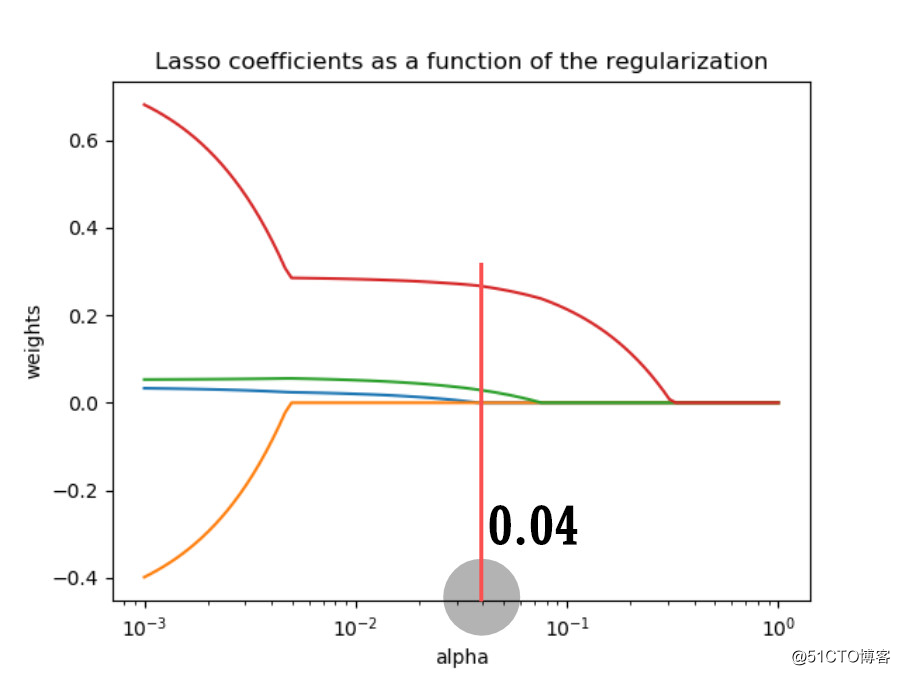发现随着正则化系数的增大，所有变量的系数会在某一阈值突降为0。

其中缘由与LASSO回归方程有关，不细说。

输出LASSO回归的变量系数。

print(lcv.coef_)

# 输出结果

[0.         0.         0.02789489 0.26549855]

发现前两个变量被筛选掉了，即年龄和收入。

为啥和岭回归的结果不一样呢？？？

/ 03 / 总结

坑留的有点多，待小F慢慢填...

相关资料获取，请点击阅读原文。

···  END  ···展开全文• 我们试着用正则化分类数据，不过除了应用正则化以外，如果能通过比较过拟合时图的状态和应用了正则化后图的状态，具体总结出正则化对模型施加了什么样的影响就更好了。 我们先构造一个函数 我们造一些向这个g(x)...

目录

一、确认训练数据

二、不应用正则化的实现

三、应用了正则化的实现

# 一、确认训练数据

我们试着用正则化分类数据，不过除了应用正则化以外，如果能通过比较过拟合时图的状态和应用了正则化后图的状态，具体总结出正则化对模型施加了什么样的影响就更好了。

我们先构造一个函数

我们造一些向这个g(x) 加入了一点噪声的训练数据，然后将它们画成图

import numpy as np
import matplotlib.pyplot as plt
#真正的函数
def g(x):
return 0.1 * (x ** 3 + x ** 2 + x)
#随意准备一些向真正的函数加入了一点噪音的训练数据
train_x = np.linspace(-2, 2, 8)
train_y = g(train_x) + np.random.randn(train_x.size) * 0.05
#绘图确认
x = np.linspace(-2, 2, 100)
plt.plot(train_x, train_y, 'o')
plt.plot(x, g(x), linestyle = 'dashed')
plt.ylim(-1, 2)
plt.show()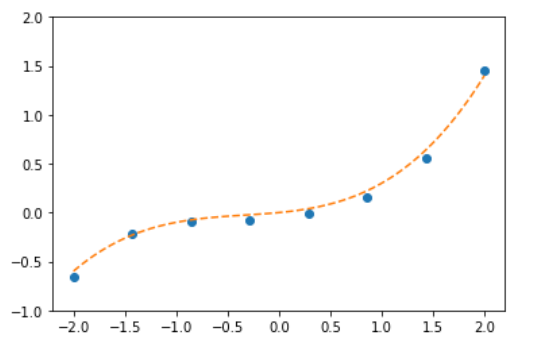虚线就是正确的g(x) 的图形，圆点就是加入了一点噪声的训练数据，我先准备了8 个数据。假设我们用10 次多项式来学习这个训练数据。首先编写从创建训练数据的矩阵到预测函数的定义为止的代码。

# 标准化
mu = train_x.mean()
sigma = train_x.std()
def standardize(x):
return (x - mu) / sigma
train_z = standardize(train_x)
# 创建训练数据的矩阵
def to_matrix(x):
return np.vstack([
np.ones(x.size),
x,
x ** 2,
x ** 3,
x ** 4,
x ** 5,
x ** 6,
x ** 7,
x ** 8,
x ** 9,
x ** 10,
]).T
X = to_matrix(train_z)
# 参数初始化
theta = np.random.randn(X.shape)
# 预测函数
def f(x):
return np.dot(x, theta)

# 二、不应用正则化的实现

那我们就开始实现学习部分吧。首先是不应用正则化的状态。η 值和学习的结束条件是根据我之前多次尝试的结果来决定的。

# 目标函数
def E(x, y):
return 0.5 * np.sum((y - f(x)) ** 2)
# 学习率
ETA = 1e-4
# 误差
diff = 1
# 重复学习
error = E(X, train_y)
while diff > 1e-6:
theta = theta - ETA * np.dot(f(X) - train_y, X)
current_error = E(X, train_y)
diff = error - current_error
error = current_error
# 对结果绘图
z = standardize(x)
plt.plot(train_z, train_y, 'o')
plt.plot(z, f(to_matrix(z)))
plt.show()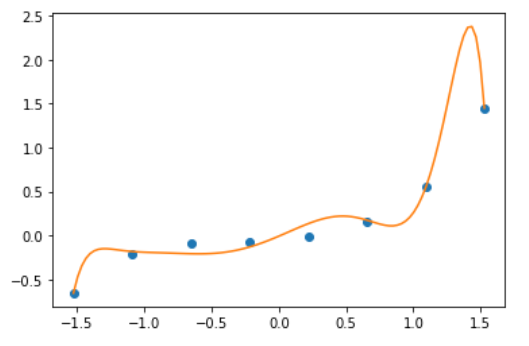这就是发生了过拟合的状态。由于参数的初始值是随机数，所以每次执行时这个图的形状都不一样。但是，从该图中也能看出它与g(x) 相差很远。

# 三、应用了正则化的实现

# 保存未正则化的参数，然后再次参数初始化
theta1 = theta
theta = np.random.randn(X.shape)
# 正则化常量
LAMBDA = 1
# 误差
diff = 1
# 重复学习（包含正则化项）
error = E(X, train_y)
while diff > 1e-6:
# 正则化项。偏置项不适用正则化，所以为0
reg_term = LAMBDA * np.hstack([0, theta[1:]])
# 应用正则化项，更新参数
theta = theta - ETA * (np.dot(f(X) - train_y, X) + reg_term)
current_error = E(X, train_y)
diff = error - current_error
error = current_error
# 对结果绘图
plt.plot(train_z, train_y, 'o')
plt.plot(z, f(to_matrix(z)))
plt.show()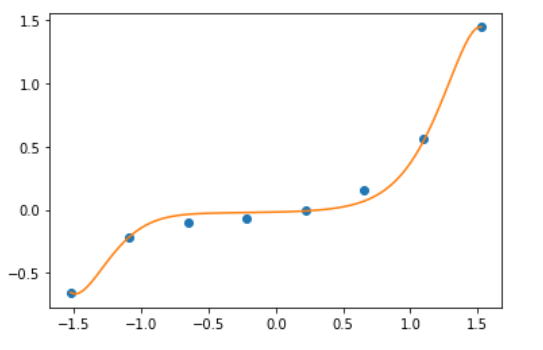为了便于比较，我把未应用和应用了正则化这两种情况展示在一张图上了。虚线是未应用正则化的情况，而实线是应用了正则化的情况。

# 保存应用了正则化的参数
theta2 = theta
plt.plot(train_z, train_y, 'o')
# 画出未应用正则化的结果
theta = theta1
plt.plot(z, f(to_matrix(z)), linestyle='dashed')
# 画出应用了正则化的结果
theta = theta2
plt.plot(z, f(to_matrix(z)))
plt.show()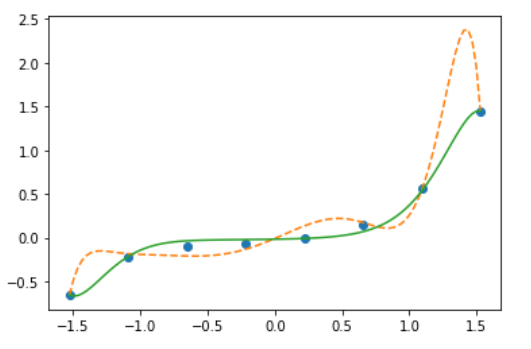通过上述对比实验，我们不难发现，应用了正则化的效果要更好。

展开全文机器学习 分类算法
• L2正则化python实现案例(附代码)，含图形展示，对于正则化理解又直观帮助
• 关于数据预处理的几个概念 归一 (Normalization)： 属性缩放到一个指定的最大和最小值（通常是1-0）之间，这可以通过preprocessing.MinMaxScaler类实现。 常用的最小最大规范方法(x-min(x))/(max(x)-min(x)...
• 数据归一化、标准化、正则化1、归一化是将数据放缩到0~1区间，利用公式(x-min)/(max-min)2、标准化将数据转化为标准的正态分布，均值为0，方差为13、正则化正则化的主要作用是防止过拟合，对模型添加正则化项可以...
• 正则化有助于克服过度拟合模型的问题。过度拟合是偏差和方差平衡的概念。如果过度拟合，机器学习模型将具有较低的准确性。...不同的正则化技术：L1和L2正则化Dropout数据增强提前停止(Early stoppi...
• import pandas as pd import re #创建DataFrame df1 = pd.DataFrame([['2015-03-24'],['2011-07-12'],['2010-02-08']]) #使用apply()和lambda进行提取 df1 = df1.apply(lambda x:re.findall('\d+-(\d+)-\d+',x)...
• ## python正则化表达式

千次阅读 2019-02-04 07:59:32
• python使用正则化检查字符串的起始和结束字符相同 #python使用正则化检查字符串的起始和结束字符相同 # Python program to check if a string starts # and ends with the same character # import re ...字符串 机器学习
• ## python正则化

千次阅读 2017-06-12 14:18:15
python浅谈正则的常用方法覆盖范围70%以上 上一次很多朋友写文字屏蔽说到要用正则表达 ，其实不是我不想用（我正则用得不是很多，看过我之前爬虫的都知道，我直接用BeautifulSoup的网页标签去找内容...
• 数据正规（data normalization）是将数据的每个样本（向量）变换为单位范数的向量，各样本之间是相互独立的．其实际上，是对向量中的每个分量值除以正规因子．常用的正规因子有 L1, L2 和 Max．假设，对长度为...
• 【课程简介】 清华大学出品的Python课件，非常适合Python新手，也适合...清华大学精品Python学习PPT课件-第12章 Python项目实战：数据可视.pptx 清华大学精品Python学习PPT课件-第13章 Python项目实战：数据分析.pptx
• 说说正则表达式字符串前的r前缀 re模块综合应用实例 参考文档 提示：由于该站对MARKDOWN的表格支持的不是很好，所以本文中的表格均以图片的形式提供，大家如果看着比较模糊，可以放大来看或下载图片在本地查看。...前端 后端
• 正则化实例 还是使用前面“比萨饼价格预测”的例子： （1）L1 范数正则化—— Lasso 模型在 4 次多项式特征上的拟合表现 # 输入训练样本的特征以及目标值，分别存储在变量 X_train 与 y_train 之中 X_train = [, ...机器学习
• 4. 正则化，增加数据，减少模型参数5. 可以从两方面回答，一个是增加约束条件，通过KKT条件，等价于增加正则化。第二，从概率论的角度。L1正则化是先验概率为拉普拉斯分布；L2正则是先验概率为高斯分布...
• python正则表达式筛选网页内容的方法发布时间：2020-09-02 13:47:51来源：亿速云阅读：101作者：小新这篇文章主要介绍了python正则表达式筛选网页内容的方法，具有一定借鉴价值，需要的朋友可以参考下。...
• 而随着训练次数增多，它慢慢找出了数据的模式，能在尽可能多地拟合数据点的同时反映数据趋势，这时它是一个性能较好的模型。在这基础上，如果我们继续训练，那模型就会进一步挖掘训练数据中的细节和噪声，为了拟合...
• I am having some trouble wrapping my head around Python regular expressions to come up with a regular expression to extract specific values.The page I am trying to parse has a number of productIds whi...
• 在看完大佬们写的几篇很有启示的文章后，链接：机器学习中常常提到的正则化到底是什么意思？​www.zhihu.comElasticNet回归及机器学习正则化_人工智能_曾时明月-CSDN博客​blog.csdn.net以下为个人对正则化...
• 为了解决神经网络过拟合问题，相较于添加数据量的难度于开销，正则化应是我们的首选方法。本实验利用Python，分别实现了L2正则化和dropout两种方法。 L2正则化 L2正则化是解决过拟合的常用方法之一。 实现L2正则化...人工智能 神经网络 机器学习 深度学习
• 正则表达式'\d+'表示提取所有数字(至少一位数字或以上)。 import pandas as pd import re data = pd.read_csv('数据') riqi = data['日期'].apply(lambda x: re.findall(r'\d+', x)) #riqi得到的是所有的日期数据...
• 1.处理文本数据的日期格式统一 text = "2015年8月31日，衢州元立金属制品有限公司仓储公司（以下简称元立仓储公司）成品仓库发生一起物体打击事故，造成直接经济损失95万元。" text1 = "2015/12/28下达行政处罚...
• ## 正则化逻辑回归-python实现

千次阅读 热门讨论 2021-08-29 15:17:41
正则化逻辑回归-python实现机器学习前言一、基础概念二、构造损失函数三、梯度下降法求解最小值四、正则化逻辑回归总结 基于吴恩达机器学习的习题 逻辑回归github地址 前言 机器学习是从人工智能中产生的一个重要...机器学习
• Python使用正则表达式爬取网页信息一、正则表达式是什么?二、实战项目1.访问链接2.项目源代码 一、正则表达式是什么? 概念: 正则表达式是对字符串操作的一种逻辑公式，就是用事先定义好的一些特定字符、及这些特定...
• 对特征进行一定的处理，可以提升算法模型的结果，主要分为归一化，标准化，正则化python的sklearn.preprocessing提供了相应的方法，使用起来非常方便。标准化
• 在pdf转为文本的时候，经常会多出空格，影响数据观感，因此需要去掉文本中多余的空格，而文本中的英文之间的正常空格需要保留，输入输出如下：input：我今天 赚了 10 个亿，老百姓very happy。output：我今天赚了10......